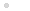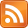### My CPU

Homebrew CPU construction / házi készítésű processzor

### Feedek## FunCPU - Parenthesis, Precedence

### 2014.07.22. 17:03 Budapesti álmodozó

When evaluating expressions, the CPU must exactly know how to evaluate an expression, what function/expression parts should be evaluated first, next, and last etc. Therefore the representation of expressions and functions must be unambigous with respect to parenthesis and priorities.

In mathematics, by convention some functions take precedence over other functions (e.g. multiplication has higher precedence than addition). To override such precedence, parenthesis can be used. See, for instance:

4+5*6 = 34
(4+5)*6=45

It is natural to think of introducing then parenthesis to make the meaning of expressions exact. However, this could result in additional complexity for evaluation, not to mention the fact, that parenthesis must be encoded by extra symbols.

Something simple was required, which could be implemented with the minimum hardware. So I have decided on using the Polish prefix notation. The above two expressions thus become the following respectively:
+ 4 * 5 6 = 34
* 6 + 4 5 = 45

As long as the arity of functions are fixed and known in advance, this notation represents the expressions unambiguously.

To implement this in hardware a simple 4 bit counter is used. The scope of a function then can be determined by using this counter as follows:

• Counter initial value will be the arity of the function of which scope is to be determined.
• If a constant is found, then counter is decreased by one.
• If a function with arity n is found, then the counter is increased by n-1.
• If counter reaches zero, then this means it was the last symbol in the function scope.

The following example demonstrates the use of the counter to determine the scope of the first „fac":

if 2 fac + fac 2 fac 1 if ... ac=1
if 2 fac + fac 2 fac 1 if ... ac=2 =1-1+2
if 2 fac + fac 2 fac 1 if ... ac=2=2-1+1
if 2 fac + fac 2 fac 1 if ... ac=1 =2-1
if 2 fac + fac 2 fac 1 if ... ac=1=2-1+1
if 2 fac + fac 2 fac 1 if ... ac=0=1-1

Please note that actually this is achieved in hardware a little bit differently. As arity is encoded in two’s complement, counter is increased by one, if a constant is found, and it is decreased when a function in scope is encountered. Also its initial value stores the function arity in two’s complement.

The choice of a 4 bit counter seems to be rather limited, but it is in balance with other hardware figures (memory, registers, etc.). Given a function f with 4 arguments, due to the 4 bit limitation the term
f(f(f(f(... is still fine (counter=4+3+3+3=13), but
f(f(f(f(f(... cannot be evaluated (counter=4+3+3+3+3=16), however,
f(f(f(f(const,f(... is ok again (4+3+3+3-1+3=15).

### Címkék: functional programmingFunCPUHomebrew CPUparenthesisoperator precedence